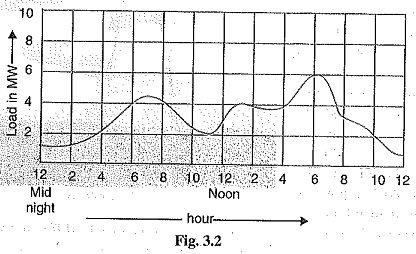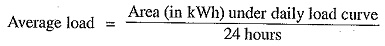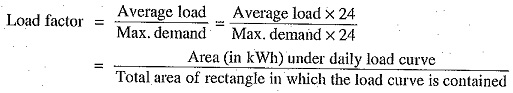## Load Curve in Power Plant:

The Load Curve in Power Plant showing the variation of load on the power station with respect to (wet) time is known as a load curve.

The load on a power station is never constant; it varies from time to time. These load variations during the whole day (i.e., 24 hours) are recorded half-hourly or hourly and are plotted against time on the graph. The curve thus obtained is known as daily load curve as it shows the variations of load cart.. time during the day. Fig. 3.2. shows a typical daily Load Curve in Power Plant. It is clear that load on the power station is varying, being maximum at 6 P.M. in this case. It may be seen that load curve indicates at a glance the general character of the load that is being imposed on the plant. Such a clear representation cannot be obtained from tabulated figures.

The monthly load curve can be obtained from the daily load curves of that month. For this purpose, average values of power over a month at different times of the day are calculated and then plotted on the graph. The monthly load curve is generally used to fix the rates of energy. The yearly load curve is obtained by considering the monthly load curves of that particular year. The yearly Load Curve in Power Plant is generally used to determine the annual load factor.The daily Load Curve in Power Plant have attained a great importance in generation as they supply the following information readily

1.The daily load curve shows the variations of load on the power station during different hours of the day.

2.The area under the daily load curve gives the number of units generated in the day. Units generated/day = Area (in kWh) under daily load curve.

3.The highest point on the daily load curve represents the maximum demand on the station on that day.

4.The area under the daily load curve divided by the total number of hours gives the average load on the station in the day.5.The ratio of the area under the load curve to the total area of rectangle in which is contained gives the load factor.6.The load curve helps in selecting the size and number of generating units.

7.The load curve helps in preparing the operation schedule of the station.

Scroll to Top